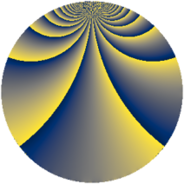# Properties

 Label 260.2.oLevel $260$ Weight $2$ Character orbit 260.o Rep. character $\chi_{260}(27,\cdot)$ Character field $\Q(\zeta_{4})$ Dimension $72$ Newform subspaces $1$ Sturm bound $84$ Trace bound $0$

# Related objects

## Defining parameters

 Level: $$N$$ $$=$$ $$260 = 2^{2} \cdot 5 \cdot 13$$ Weight: $$k$$ $$=$$ $$2$$ Character orbit: $$[\chi]$$ $$=$$ 260.o (of order $$4$$ and degree $$2$$) Character conductor: $$\operatorname{cond}(\chi)$$ $$=$$ $$20$$ Character field: $$\Q(i)$$ Newform subspaces: $$1$$ Sturm bound: $$84$$ Trace bound: $$0$$

## Dimensions

The following table gives the dimensions of various subspaces of $$M_{2}(260, [\chi])$$.

Total New Old
Modular forms 92 72 20
Cusp forms 76 72 4
Eisenstein series 16 0 16

## Trace form

 $$72 q - 8 q^{6} - 12 q^{8} + O(q^{10})$$ $$72 q - 8 q^{6} - 12 q^{8} - 8 q^{10} - 8 q^{12} - 8 q^{16} + 28 q^{18} - 16 q^{21} - 8 q^{22} - 20 q^{28} - 32 q^{30} - 40 q^{32} + 16 q^{33} + 32 q^{36} - 12 q^{38} - 8 q^{40} - 40 q^{42} - 8 q^{46} + 60 q^{48} + 40 q^{50} + 8 q^{52} - 48 q^{53} + 8 q^{56} - 60 q^{58} + 20 q^{60} - 64 q^{61} + 60 q^{62} + 8 q^{66} - 16 q^{68} - 60 q^{70} + 40 q^{72} - 16 q^{73} - 72 q^{76} + 48 q^{77} - 20 q^{80} + 8 q^{81} - 12 q^{82} + 48 q^{85} + 48 q^{86} + 12 q^{88} + 44 q^{90} - 36 q^{92} + 16 q^{93} + 32 q^{96} - 80 q^{97} - 32 q^{98} + O(q^{100})$$

## Decomposition of $$S_{2}^{\mathrm{new}}(260, [\chi])$$ into newform subspaces

Label Dim $A$ Field CM Traces $q$-expansion
$a_{2}$ $a_{3}$ $a_{5}$ $a_{7}$
260.2.o.a $72$ $2.076$ None $$0$$ $$0$$ $$0$$ $$0$$

## Decomposition of $$S_{2}^{\mathrm{old}}(260, [\chi])$$ into lower level spaces

$$S_{2}^{\mathrm{old}}(260, [\chi]) \cong$$ $$S_{2}^{\mathrm{new}}(20, [\chi])$$$$^{\oplus 2}$$